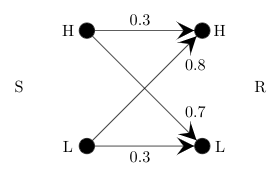Related Articles
GATE | GATE CS 2021 | Set 1 | Question 64
• Last Updated : 06 Apr, 2021

A sender (S) transmits a signal, which can be one of the two kinds: H and L with probabilities 0.1 and 0.9 respectively, to a receiver (R)
In the graph below, the weight of edge (u,v) is the probability of receiving v when u is transmitted, where u,v∈{H,L}. For example, the probability that the received signal is L given the transmitted signal was H, is 0.7.If the received signal is H, the probability that the transmitted signal was H (rounded to 2 decimal places) is __________.
(A) 0.04
(B) 0.03
(C) 0.75
(D) 1

Answer: (A)

Explanation: If the received signal is H, the probability that the transmitted signal was H,
= (0.1*0.3) / (0.1*0.3 + 0.9*0.8)
= 0.03 / 0.75
= 0.04

Quiz of this QuestionMy Personal Notes arrow_drop_up